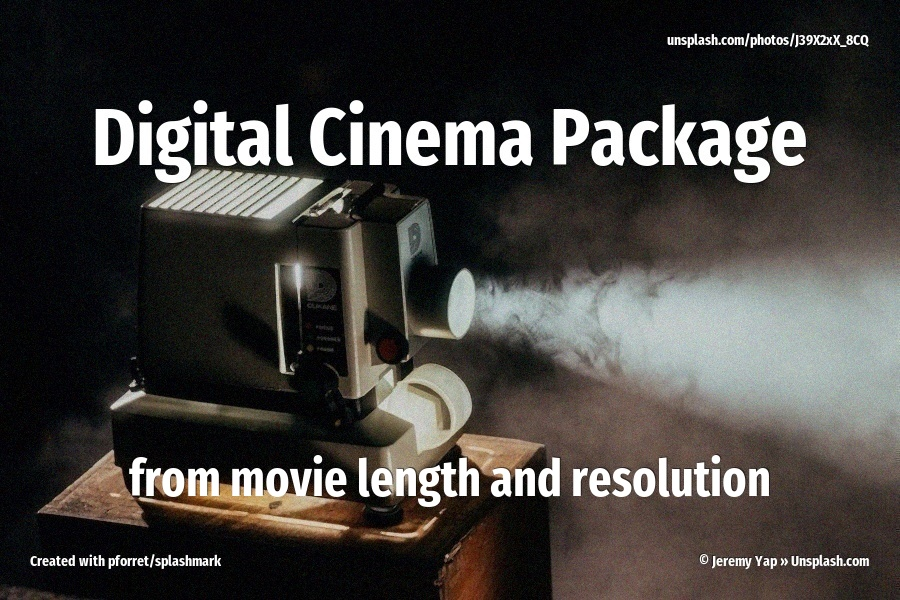# Calculate DCP size from movie length and resolution

To calculate the estimated size of a Digital Cinema Package (DCP) for cinema projection, you need to consider the image size and movie length. The DCP size is primarily determined by the image data and audio tracks.Here’s the formula to estimate the DCP size:

DCP Size = (Image Size in Megapixels × Bit Depth × Frame Rate × Movie Length in seconds * Compression rate)

• Image Size: The image size is typically expressed in megapixels (MP). For example, if the image resolution is 2K (2048x1080 pixels), the image size would be approximately 2.2 MP. If it’s 4K (4096x2160 pixels), the image size would be around 8.8 MP.
• Bit Depth: The bit depth represents the number of bits used to store color information per pixel. It is usually 10 bits or 12 bits per channel (color), which means each pixel can store a range of 1024 or 4096 colors per channel.
• Frame Rate: The frame rate indicates the number of frames shown per second in the movie. Common frame rates for cinema projection are 24 frames per second (fps) or 48 fps for higher frame rate productions.
• Movie Length: The movie length is measured in seconds.
• Compression rate: can be chosen in function of the required image quality/file size. Max bitrate is 250Mbps, and it can go as low as 50 Mbps.

Let’s take an example calculation:

Image Size: 4K (4096x2160 pixels) - Approximately 8.8 MP Bit Depth: 10 bits per channel (so 30 bits per pixel) Frame Rate: 24 frames per second (fps) Movie Length: 120 minutes (7,200 seconds)

The theoretical uncompressed size would be:

4096 x 2160 = 8.85 megapixels

filesize of 1 frame = 8.85 megapixels x 30 bits = 265 Mbit = 33,18 MB

120 minutes = 7200 seconds = 172,800 frames

uncompressed size = 172,800 x 33,18 MB = 5,73 TB

And then you can apply a compression rate to that. Typically, DCP packages will be compressed with JPEG2000 to somewhere between 100 and 250 Mbps. So that would be a compression rate from 21:1 up to 50:1.

``````Total bitrate = 250Mbps = 31.25MB/s
Video bitrate (without audio) = 243.09Mbps = 30.39MB/s
per frame: 1.27MB/frame
compression factor: 4.77% or ±21:1
``````

So the final DCP size would be

VIDEO: 172800 frames x 1,27 MB = 219,456 MB = 219 GB

AUDIO: 7200 seconds x 6 channels x 24 bits x 48 kHz = 6,22GB

TOGETHER: 225 GB